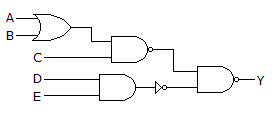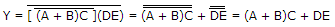# Electronics and Communication Engineering - Digital Electronics

26.

In the given figure, Y =A. (A + B)C + DE B. AB + C(D + E) C. (A + B)C + D + E D. none of the above

Explanation:.

27.

Assertion (A): The access time of memory is lowest in the case of DRAM

Reason (R): DRAM uses refreshing cycle.

 A. Both A and R are correct and R is correct explanation of A B. Both A and R are correct but R is not correct explanation of A C. A is true, R is false D. A is false, R is true

Explanation:

DRAM has lower speed than SRAM.

28.

In a 3 input NOR gate, the number of states in which output is 1 equals

 A. 1 B. 2 C. 3 D. 4

Explanation:

Only one input, i.e., A = 0, B = 0 and C = 0 gives 1 as output.

29.

Assertion (A): Even if TTL gates and CMOS gates used in a realization have the same power supply of + 5 V, suitable circuit is needed to interconnect them

Reason (R): VOH, VOL, VIH and VIL of a TTL gave are respectively 2.4, 0.4, 2 and 0.8 V respectively. If supply voltage is + 5 V. VIL and VIH for CMOS gate for the supply voltage of + 5 V are 1.5 V and 3.5 V respectively.

 A. Both A and R are correct and R is correct explanation of A B. Both A and R are correct but R is not correct explanation of A C. A is true, R is false D. A is false, R is true

Explanation:

Interfacing is necessary and interfacing depends on gate parameters like VOH, VOL, IIH, IIL.

30.

The series 54 H/74 H denotes

 A. Standard TTL B. High speed TTL C. Low Power TTL D. High Power TTL